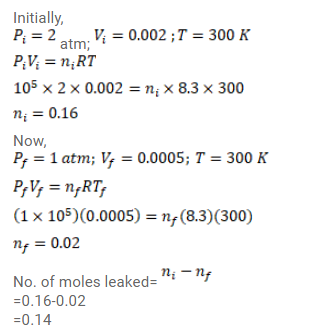# Use R=8.3 J/mol-K wherever required. Air is pumped into the tubes of a cycle rickshaw at a pressure of 2 atm.Question:

Use $\mathrm{R}=8.3 \mathrm{~J} / \mathrm{mol}-\mathrm{K}$ wherever required.

Air is pumped into the tubes of a cycle rickshaw at a pressure of 2 atm. The volume of each tube at this pressure is $0.002^{m^{3}}$. One of the tubes gets punctured and the volume of the tube reduces to $0.0005^{m^{3}}$. How many moles of air have leaked out? Assume that the temperature remains constant at $300 \mathrm{~K}$ and that the air behaves as an ideal gas.

Solution: